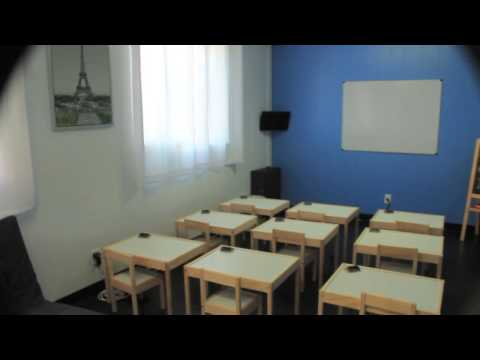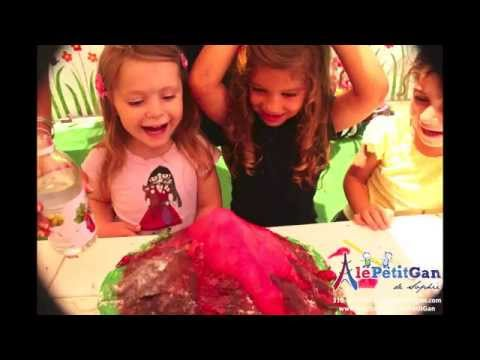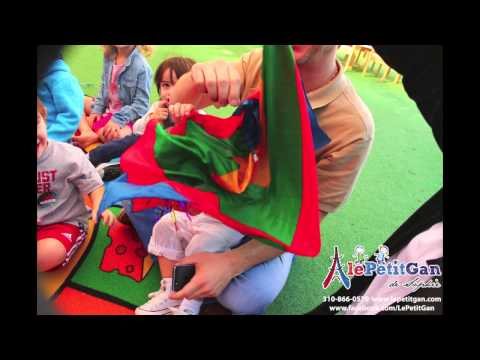First 100 Days of School 2015-2016

``````/* <![CDATA[ */
\$(function(){
var width = \$(window).innerWidth();
var setwidth = 640;
var ratio = 0.75;
var height = 480;
if(width < setwidth)
{
height = Math.floor(width * 0.75);
//console.log("device width "+width+", set width "+640+", ratio "+0.75+", new height "+ height);
var new_url = wpvl_paramReplace('width', link, width);
var new_url = wpvl_paramReplace('height', new_url, height);
\$("a#5d2f576a0133a").attr('href', new_url);
//console.log(new_url);
}
});
});
/* ]]&gt; */
``````

First 100 Days of School 2014-2015

``````/* <![CDATA[ */
\$(function(){
var width = \$(window).innerWidth();
var setwidth = 640;
var ratio = 0.75;
var height = 480;
if(width < setwidth)
{
height = Math.floor(width * 0.75);
//console.log("device width "+width+", set width "+640+", ratio "+0.75+", new height "+ height);
var new_url = wpvl_paramReplace('width', link, width);
var new_url = wpvl_paramReplace('height', new_url, height);
\$("a#5d2f576a0162b").attr('href', new_url);
//console.log(new_url);
}
});
});
/* ]]&gt; */
``````

LOVE MONTH

``````/* <![CDATA[ */
\$(function(){
var width = \$(window).innerWidth();
var setwidth = 640;
var ratio = 0.75;
var height = 480;
if(width < setwidth)
{
height = Math.floor(width * 0.75);
//console.log("device width "+width+", set width "+640+", ratio "+0.75+", new height "+ height);
var new_url = wpvl_paramReplace('width', link, width);
var new_url = wpvl_paramReplace('height', new_url, height);
\$("a#5d2f576a0190e").attr('href', new_url);
//console.log(new_url);
}
});
});
/* ]]&gt; */
``````

Talia’s 3rd Birthday

``````/* <![CDATA[ */
\$(function(){
var width = \$(window).innerWidth();
var setwidth = 640;
var ratio = 0.75;
var height = 480;
if(width < setwidth)
{
height = Math.floor(width * 0.75);
//console.log("device width "+width+", set width "+640+", ratio "+0.75+", new height "+ height);
var new_url = wpvl_paramReplace('width', link, width);
var new_url = wpvl_paramReplace('height', new_url, height);
\$("a#5d2f576a01bfe").attr('href', new_url);
//console.log(new_url);
}
});
});
/* ]]&gt; */
``````

Eli’s 3rd Birthday

``````/* <![CDATA[ */
\$(function(){
var width = \$(window).innerWidth();
var setwidth = 640;
var ratio = 0.75;
var height = 480;
if(width < setwidth)
{
height = Math.floor(width * 0.75);
//console.log("device width "+width+", set width "+640+", ratio "+0.75+", new height "+ height);
var new_url = wpvl_paramReplace('width', link, width);
var new_url = wpvl_paramReplace('height', new_url, height);
\$("a#5d2f576a01ee0").attr('href', new_url);
//console.log(new_url);
}
});
});
/* ]]&gt; */
``````

Kindergarten Class

``````/* <![CDATA[ */
\$(function(){
var width = \$(window).innerWidth();
var setwidth = 640;
var ratio = 0.75;
var height = 480;
if(width < setwidth)
{
height = Math.floor(width * 0.75);
//console.log("device width "+width+", set width "+640+", ratio "+0.75+", new height "+ height);
var new_url = wpvl_paramReplace('width', link, width);
var new_url = wpvl_paramReplace('height', new_url, height);
\$("a#5d2f576a021b0").attr('href', new_url);
//console.log(new_url);
}
});
});
/* ]]&gt; */
``````

Summer Camp 2014

``````/* <![CDATA[ */
\$(function(){
var width = \$(window).innerWidth();
var setwidth = 640;
var ratio = 0.75;
var height = 480;
if(width < setwidth)
{
height = Math.floor(width * 0.75);
//console.log("device width "+width+", set width "+640+", ratio "+0.75+", new height "+ height);
var new_url = wpvl_paramReplace('width', link, width);
var new_url = wpvl_paramReplace('height', new_url, height);
\$("a#5d2f576a02493").attr('href', new_url);
//console.log(new_url);
}
});
});
/* ]]&gt; */
``````

Edens 3rd Birthday

``````/* <![CDATA[ */
\$(function(){
var width = \$(window).innerWidth();
var setwidth = 640;
var ratio = 0.75;
var height = 480;
if(width < setwidth)
{
height = Math.floor(width * 0.75);
//console.log("device width "+width+", set width "+640+", ratio "+0.75+", new height "+ height);
var new_url = wpvl_paramReplace('width', link, width);
var new_url = wpvl_paramReplace('height', new_url, height);
\$("a#5d2f576a02775").attr('href', new_url);
//console.log(new_url);
}
});
});
/* ]]&gt; */
``````

Valentines Heart Project

``````/* <![CDATA[ */
\$(function(){
var width = \$(window).innerWidth();
var setwidth = 640;
var ratio = 0.75;
var height = 480;
if(width < setwidth)
{
height = Math.floor(width * 0.75);
//console.log("device width "+width+", set width "+640+", ratio "+0.75+", new height "+ height);
var new_url = wpvl_paramReplace('width', link, width);
var new_url = wpvl_paramReplace('height', new_url, height);
\$("a#5d2f576a02a54").attr('href', new_url);
//console.log(new_url);
}
});
});
/* ]]&gt; */
``````

Liam’s 2nd Birthday

``````/* <![CDATA[ */
\$(function(){
var width = \$(window).innerWidth();
var setwidth = 640;
var ratio = 0.75;
var height = 480;
if(width < setwidth)
{
height = Math.floor(width * 0.75);
//console.log("device width "+width+", set width "+640+", ratio "+0.75+", new height "+ height);
var new_url = wpvl_paramReplace('width', link, width);
var new_url = wpvl_paramReplace('height', new_url, height);
\$("a#5d2f576a02d2e").attr('href', new_url);
//console.log(new_url);
}
});
});
/* ]]&gt; */
``````

Yalie’s 2nd Birthday

``````/* <![CDATA[ */
\$(function(){
var width = \$(window).innerWidth();
var setwidth = 640;
var ratio = 0.75;
var height = 480;
if(width < setwidth)
{
height = Math.floor(width * 0.75);
//console.log("device width "+width+", set width "+640+", ratio "+0.75+", new height "+ height);
var new_url = wpvl_paramReplace('width', link, width);
var new_url = wpvl_paramReplace('height', new_url, height);
\$("a#5d2f576a0300e").attr('href', new_url);
//console.log(new_url);
}
});
});
/* ]]&gt; */
``````

To Top